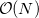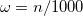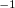Q-Chem 4.3 User’s Manual

# 4.11 Unconventional SCF Calculations

## 4.11.1 CASE Approximation

The Coulomb Attenuated Schrödinger Equation (CASE)  approximation follows from the KWIK  algorithm in which the Coulomb operator is separated into two pieces using the error function, Eq. (). Whereas in Section 4.3.4 this partition of the Coulomb operator was used to incorporate long-range Hartree-Fock exchange into DFT, within the CASE approximation it is used to attenuate all occurrences of the Coulomb operator in Eq. (4.2), by neglecting the long-range portion of the identity in Eq. (). The parameterin Eq. () is used to tune the level of attenuation. Although the total energies from Coulomb attenuated calculations are significantly different from non-attenuated energies, it is found that relative energies, correlation energies and, in particular, wavefunctions, are not, provided a reasonable value ofis chosen.

By virtue of the exponential decay of the attenuated operator, ERIs can be neglected on a proximity basis yielding a rigorousalgorithm for single point energies. CASE may also be applied in geometry optimizations and frequency calculations.

OMEGA
 Controls the degree of attenuation of the Coulomb operator.

TYPE:
 INTEGER

DEFAULT:
 No default

OPTIONS:Corresponding to, in units of bohrRECOMMENDATION:
 None

INTEGRAL_2E_OPR
 Determines the two-electron operator.

TYPE:
 INTEGER

DEFAULT:
 -2 Coulomb Operator.

OPTIONS:
 -1 Apply the CASE approximation. -2 Coulomb Operator.

RECOMMENDATION:
 Use default unless the CASE operator is desired.

## 4.11.2 Polarized Atomic Orbital (PAO) Calculations

Polarized atomic orbital (PAO) calculations are an interesting unconventional SCF method, in which the molecular orbitals and the density matrix are not expanded directly in terms of the basis of atomic orbitals. Instead, an intermediate molecule-optimized minimal basis of polarized atomic orbitals (PAOs) is used . The polarized atomic orbitals are defined by an atom-blocked linear transformation from the fixed atomic orbital basis, where the coefficients of the transformation are optimized to minimize the energy, at the same time as the density matrix is obtained in the PAO representation. Thus a PAO-SCF calculation is a constrained variational method, whose energy is above that of a full SCF calculation in the same basis. However, a molecule optimized minimal basis is a very compact and useful representation for purposes of chemical analysis, and it also has potential computational advantages in the context of MP2 or local MP2 calculations, as can be done after a PAO-HF calculation is complete to obtain the PAO-MP2 energy.

PAO-SCF calculations tend to systematically underestimate binding energies (since by definition the exact result is obtained for atoms, but not for molecules). In tests on the G2 database, PAO-B3LYP/6-311+G(2df,p) atomization energies deviated from full B3LYP/6-311+G(2df,p) atomization energies by roughly 20 kcal/mol, with the error being essentially extensive with the number of bonds. This deviation can be reduced to only 0.5 kcal/mol with the use of a simple non-iterative second order correction for “beyond-minimal basis” effects . The second order correction is evaluated at the end of each PAO-SCF calculation, as it involves negligible computational cost. Analytical gradients are available using PAOs, to permit structure optimization. For additional discussion of the PAO-SCF method and its uses, see the references cited above.

Calculations with PAOs are determined controlled by the following \$rem variables. PAO_METHOD = PAO invokes PAO-SCF calculations, while the algorithm used to iterate the PAO’s can be controlled with PAO_ALGORITHM.

PAO_ALGORITHM
 Algorithm used to optimize polarized atomic orbitals (see PAO_METHOD)

TYPE:
 INTEGER

DEFAULT:
 0

OPTIONS:
 0 Use efficient (and riskier) strategy to converge PAOs. 1 Use conservative (and slower) strategy to converge PAOs.

RECOMMENDATION:
 None

PAO_METHOD
 Controls evaluation of polarized atomic orbitals (PAOs).

TYPE:
 STRING

DEFAULT:
 EPAO For local MP2 calculations Otherwise no default.

OPTIONS:
 PAO Perform PAO-SCF instead of conventional SCF. EPAO Obtain EPAO’s after a conventional SCF.

RECOMMENDATION:
 None